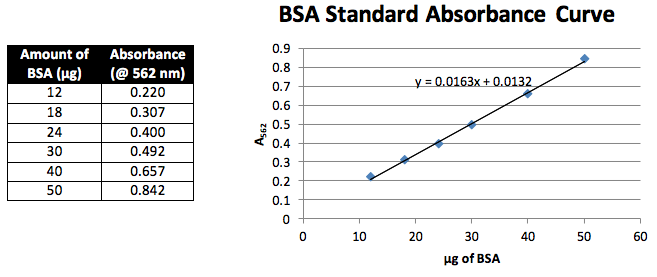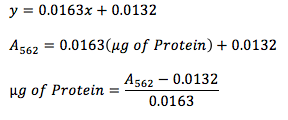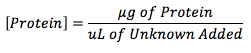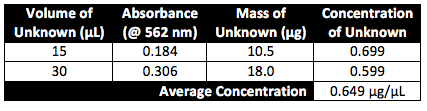# BCA Protein Concentration Assay

Objective

Utilizing Beer’s law, known concentrations of bovine serum albumin (BSA) are reacted with bicinchonic acid (BCA) to create a standard curve of absorbance vs. concentration of protein.  This curve is then utilized to determine the concentration of protein in an unknown solution.

Introduction

Beer’s law () tells us that the light absorbance (A) of a solution is proportional to the concentration of the protein (c) within the solution, the light path (b) and the extinction coefficient of the protein.  While one can measure the absorbance of proteins with ultraviolet light (280 nm), this method is inconsistent due to the absorbance differences between the three aromatic amino acids; phenylalanine, tyrosine and tryptophan.  Proteins that have higher levels of tryptophan will have a higher absorbance than those with lower levels regardless of a potentially lower protein concentration.  However, by reacting the protein with a substance to produce colorimetric substrate, absorbance can be measured within the visible spectrum at the wavelength of the color of the products.  Since the substrate reacts with the protein in the same proportions for both a standard protein and the target protein, the absorbance of both can be directly compared.

Methods

Preparing the Standards:

Seven samples were created in varying concentrations of BSA.  0, 6, 9, 12, 15, 20 and 25 µL of 2.0 mg/mL BSA were each mixed with H2O to obtain a final volume of 50 µL.  Then 950 µL of BCA reagent was added to each and the samples were then incubated at 37 oC for 20 min.  The sample with no added BSA was utilized as a blank to zero the spectrometer.  Then each entire sample was placed in a 1 cm cuvette and the absorbance at 562 nm was measured.  These values were then plotted to create our standard curve (graph 1).

Preparing the Samples:

Two samples of our unknown solution were created by mixing 15 µL and 30 µL each with enough H2O to obtain a final volume 50 µL.  To these samples, 950 µL of BCA reagent was added and the samples were then incubated at 37 oC for 20 min.  These samples were then read in the spectrometer, utilizing the same blank as the standards, measuring the absorbance at 562 nm.

Results

Standards:Equation 1The mass of protein in the unknown solution was calculated utilizing Equation 1.

Equation 2Concentration of protein in the unknown solution was calculated utilizing Equation 2.

Unknown A:Conclusion and Discussion:

Both of the experimental concentrations obtained are relatively close so the accuracy of average concentration is reasonable.  The deviation between the two values can be attributed to the fact that the same cuvette was utilized for each spectrometer reading.  Trace amounts of the previous sample present in the cuvette, particularly between the last standard and the first sample could account for the higher absorbance value and thus the higher concentration.  Our experiment deviated from the established protocol by the lengthening of the incubation time.  After 15 minutes at 37 oC, our first unknown sample (the one with 15 µL of unknown) had not yet begun to show the purple coloring.  Incubation of both the samples and the standards was lengthened until a distinct purple hue was obtained in order to ensure more accurate results.

• Share